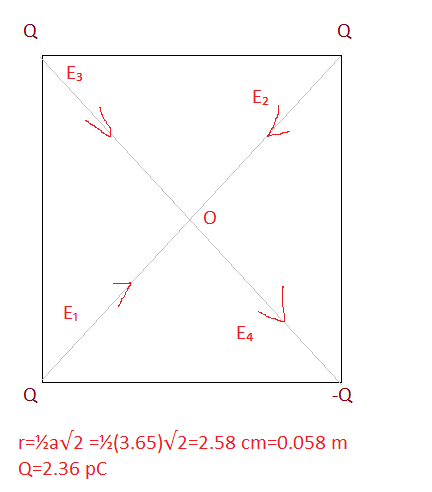# Four-point charges have the same magnitude of 2.36 10 ? 12 C and are fixed to the corners of...

## Question:

Four-point charges have the same magnitude of {eq}2.36 \times10^{-12}\; C {/eq} and are fixed to the corners of a square that is 3.65 cm on aside. Three of the charges are positive and one is negative. Determine the magnitude of the net electric field that exists at the center of the square.

## Superposition Principle:

The electric field at a point due to a number of charges in a plane is given by the vector sum of the fields produced by each charge. The field due to the point charge kept at the diagonally opposite corners of the square will be equal and opposite at the center of the square if they are like charges. And will be added if they are unlike charges.The electric field due to the two like charges at the diagonally opposite corner cancel each other as shown in figure.

The electric field due to unlike charge will add up to give the resultant electric field

{eq}E=E_3+E_4 \\ E=2E_3 \\ E= 2 \times 9 \times 10^9 \dfrac{Q}{r^2} \\ E= 2 \times 9 \times 10^9 \dfrac{2.36 \times 10^{-12}}{0.058^2} \\ E=12.628\ N/C {/eq}